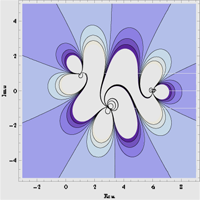March 2011
Mon Tue Wed Thu Fri Sat Sun
« Feb   Apr »
123456
78910111213
14151617181920
21222324252627
28293031

## The horizons of observability in PT-symmetric four-site quantum lattices

Miloslav ZnojilThe “Hermitizability” problem of quantum theory is explained, discussed and illustrated via the discrete-lattice cryptohermitian quantum graphs. In detail, the description of the domain ${\cal D}$ of admissible parameters is provided for the “circular-model” three-parametric quantum Hamiltonian $H$ using periodic boundary conditions. It is emphasized that even in such an elementary system the weak- and strong-coupling subdomains of ${\cal D}$ become, unexpectedly, non-empty and disconnected.

http://arxiv.org/abs/1103.4001
Quantum Physics (quant-ph); High Energy Physics – Theory (hep-th); Mathematical Physics (math-ph)

## PT-symmetry in honeycomb photonic lattices

Alexander Szameit, Mikael C. Rechtsman, Omri Bahat-Treidel, Mordechai Segev

We apply gain/loss to honeycomb photonic lattices and show that the dispersion relation is identical to tachyons – particles with imaginary mass that travel faster than the speed of light. This is accompanied by PT-symmetry breaking in this structure. We further show that the PT-symmetry can be restored by deforming the lattice.

http://arxiv.org/abs/1103.3389
Optics (physics.optics)

## On the trapping fermions with PT-symmetric potentials in the presence of position-dependent mass

Luis B. Castro

The relativistic problem of fermions subject to a PT-symmetric potential in the presence of position-dependent mass is reinvestigated. The influence of the PT-symmetric potential in the continuity equation and in the orthonormalization condition are analyzed. In addition, a misconception diffused in the literature on the interaction of neutral fermions is clarified.

http://arxiv.org/abs/1103.2460
Quantum Physics (quant-ph); High Energy Physics – Theory (hep-th); Mathematical Physics (math-ph)

## PT-symmetry breaking in complex nonlinear wave equations and their deformations

Andrea Cavaglia, Andreas Fring, Bijan BagchiWe investigate complex versions of the Korteweg-deVries equations and an Ito type nonlinear system with two coupled nonlinear fields. We systematically construct rational, trigonometric/hyperbolic, elliptic and soliton solutions for these models and focus in particular on physically feasible systems, that is those with real energies. The reality of the energy is usually attributed to different realisations of an antilinear symmetry, as for instance PT-symmetry. It is shown that the symmetry can be spontaneously broken in two alternative ways either by specific choices of the domain or by manipulating the parameters in the solutions of the model, thus leading to complex energies. Surprisingly the reality of the energies can be regained in some cases by a further breaking of the symmetry on the level of the Hamiltonian. In many examples some of the fixed points in the complex solution for the field undergo a Hopf bifurcation in the PT-symmetry breaking process. By employing several different variants of the symmetries we propose many classes of new invariant extensions of these models and study their properties. The reduction of some of these models yields complex quantum mechanical models previously studied.

http://arxiv.org/abs/1103.1832
Mathematical Physics (math-ph); High Energy Physics – Theory (hep-th); Quantum Physics (quant-ph)